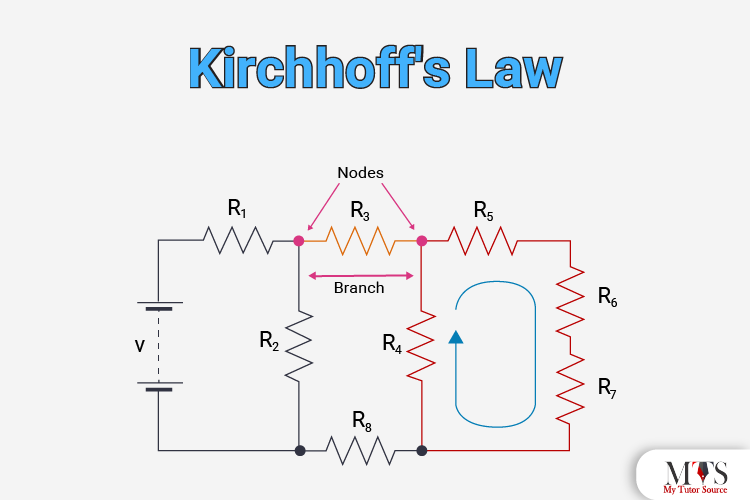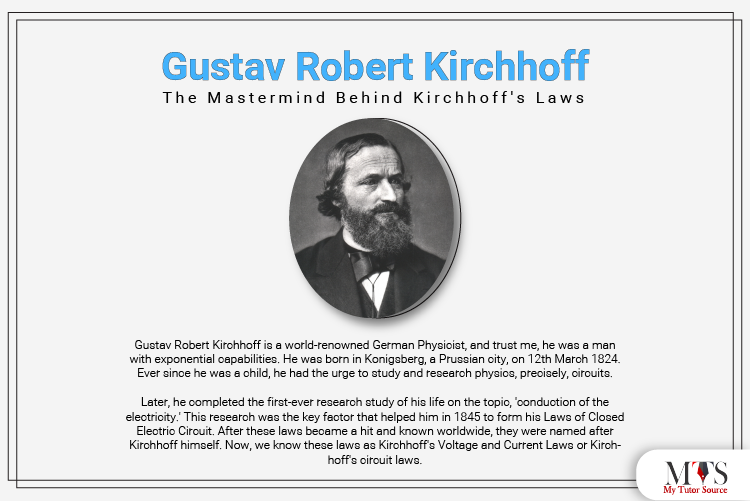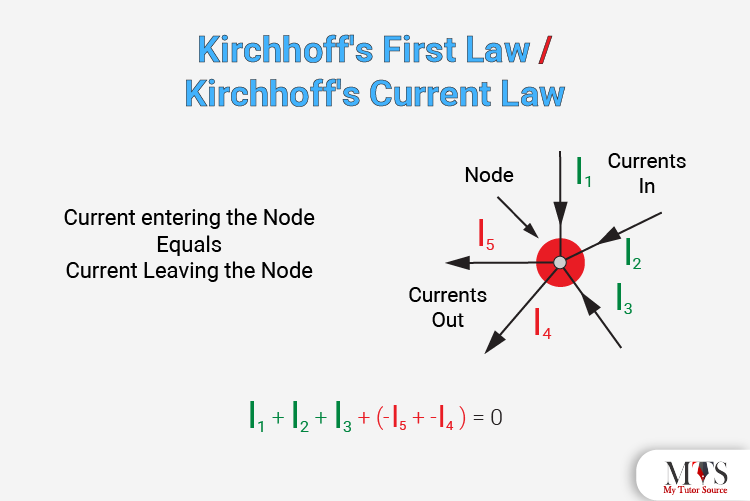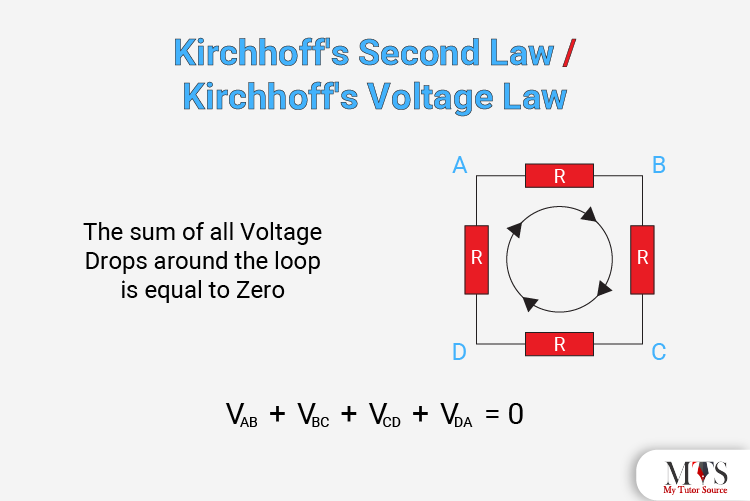# Kirchhoff's Law: Everything you need to know!Kirchhoff's laws are one of the essential parts of Physics and, obviously, a circuit. Do you know that a circuit literally works because of Kirchhoff's laws? Yes, these laws have provided us with a tool to analyze the circuits and all their components like inductors, capacitors, and indicators.

In this post, we will go through Kirchhoff's current law and Kirchhoff's voltage law in detail and how to engage them in circuit analysis.

But before that, let's go through a brief history of Kirchhoff's laws and the person who introduced them. Keep Reading!

## Gustav Robert Kirchhoff - The Mastermind Behind Kirchhoff's LawsGustav Robert Kirchhoff is a world-renowned German Physicist, and trust me, he was a man with exponential capabilities. He was born in Konigsberg, a Prussian city, on 12th March 1824. Ever since he was a child, he had the urge to study and research physics, precisely, circuits.

Later, he completed the first-ever research study of his life on the topic, 'conduction of the electricity.' This research was the key factor that helped him in 1845 to form his Laws of Closed Electric Circuit. After these laws became a hit and known worldwide, they were named after Kirchhoff himself. Now, we know these laws as Kirchhoff's Voltage and Current Laws or Kirchhoff's circuit laws.

These laws are applied to all the circuits present in this world to analyze and study them. Therefore, understanding the fundamentals and concepts of Kirchhoff's circuit laws is exceptionally essential. Learning and understanding Kirchhoff's laws is the only way to understand further how a circuit works.

Though the Kirchhoff's circuit laws are the most significant invention in the world of Electrical Engineering to date, the physicist has made some other noticeable discoveries as well! He was the first person in this world who found that an electrical impulse travelled at the speed of light. Moreover, the physicist is known to have a significant contribution to the world of spectroscopy and blackbody radiation!

## What are Kirchhoff's Circuit laws?

As we have mentioned above, Gustav Robert Kirchhoff introduced a pair of circuit laws in 1845. These laws included the concept of the conservation of energy and current inside electrical circuits. These two laws are known as Kirchhoff's voltage law and Kirchhoff's current law. These laws are used to calculate the electrical resistance of a complex network. A network can be complex when the flow of the current and AC are in different streams.

Here is a brief detail about what these laws mainly state:

• Kirchhoff's current law also goes by the names Kirchhoff's first law and Kirchhoff's junction rule. Now you must be thinking, what is the junction rule, right? Let us explain. The junction rule states that all the currents in a junction are equal to the sum of all the currents outside the junction.
• Kirchhoff's voltage law also goes by the names of Kirchhoff's second law and Kirchhoff's loop rule. According to this rule, the sum of all the voltages in a circuit around the loop equals null.
Now, let us go through these two laws in detail!

### Kirchhoff's First Law / Kirchhoff's Current Law

Kirchhoff's current law states that the,

"total current or charge entering a junction or node is exactly equal to the charge leaving the node as it has no other place to go except to leave, as no charge is lost within the node."

In simpler words, the algebraic sum of every current leaving and entering the node should be null. For example, I(exiting) + I(entering) = 0. This property is also known as the Conversion of Charge.

Here is a visual explanation for you to understand better the concept of Kirchhoff's current law:This image shows that the currents I1, I2, and I3 entering the node are positive. However, the currents I4 and I5 leaving the node are negative. Thus, we can write the equation as follows:

I1 + I2 + I3 – I4 – I5 = 0

### Kirchhoff's Second Law / Kirchhoff's Voltage Law

Kirchhoff's second law / Kirchhoff's voltage law states that:

“In any closed-loop network, the total voltage around the loop is equal to the sum of all the voltage drops within the same loop which is also equal to zero.”

In simpler words, the algebraic sum of all voltage drops inside the loop must be equal to zero. This property is also known as the Conversion of Energy. Moreover, we can use this Kirchhoff's law for analyzing a series circuit.

Here is a visual representation for you to understand this Kirchhoff's law better:No matter where you start in the loop, the sum of all the voltage drops will always be equal to zero. Also, the loop will always continue in the same direction, either clockwise or anti-clockwise. If the direction is not the same, the sum won't be equal to zero.

## Common DC Circuit Terms

We knew you might not understand a few terms we mentioned above while explaining Kirchhoff's Circuit laws. Therefore, here is a brief definition of all the common terms:

• Circuit: A circuit is a closed-loop conducting path through which the electric current flows.
• Path: A path is a single line that connects sources and elements.
• Node: A node is known as a connection, junction, or terminal inside a circuit. It is present where two or more elements of the circuit are joined. A nod is usually represented by a dot.
• Branch: A branch can be a single component or a group of components connected between two nodes. Examples of components can be a resistor or a source.
• Loop: The loop is also a closed path present inside a circuit. In this path, no node or circuit element can come across more than once.

## Application of Kirchhoff's Circuit Laws

Kirchhoff's circuit laws are responsible for enabling the currents and voltages found in a circuit. That is how they analyze the circuit. To have a better idea, here is the basic procedure of how to use Kirchhoff's circuit laws:

1. First of all, you need to name all the voltages and resistances if not already given. For example, V1, V2, V3, R1, R2, etc.
2. You need to assign a clockwise or anti-clockwise current to every branch.
3. Then, you need to label every branch with a branch current. For example, I1, I2, and I3.
4. After that, you need to find out Kirchhoff's first law equation for every node.
5. Then, find out Kirchhoff's second law equation for all the independent loops inside the circuit.
6. Find unknown currents by using linear simultaneous equations.

## Conclusion

The concept of Kirchhoff's laws may be a bit tricky to absorb, but once you understand it, you will know how interesting and easy it is. Also, it is one of the most important concepts of Physics, so don't think you can just forego it.

If you are still having difficulties understanding Kirchhoff's law even after this post, you can always request a professional tutor from our website. Our highly qualified and experienced tutors will clear all your concepts. Don't hesitate, we are just a click away!

## Frequently Asked Questions

What is the Kirchhoff's Current Law?
Kirchhoff's current law states that the total current or charge entering a junction or node is exactly equal to the charge leaving the node.

What is another name for Kirchhoff's first law?
Kirchhoff's first law is also known as Kirchhoff's current law.

Who introduced Kirchhoff's laws?
Kirchhoff's laws were introduced by a German physicist named Gustav Robert Kirchhoff.

What is another name for Kirchhoff's second law?
Kirchhoff's second law is also known as Kirchhoff's voltage law.

### Find Top Tutors in Your AreaWith over 3 years of experience in teaching, Chloe is very deeply connected with the topics that talk about the educational and general aspects of a student's life. Her writing has been very helpful for students to gain a better understanding of their academics and personal well-being. I’m also open to any suggestions that you might have! Please reach out to me at chloedaniel402 [at] gmail.com